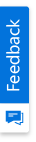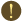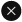We use cookies to give you the best experience on our website. If you continue to browse, then you agree to our privacy policy and cookie policy.Unfortunately, activation email could not send to your email. Please try again.# ASP.NET MVC Radar Chart - High Performance Chart

## Overview

The ASP.NET MVC Radar Chart visualizes data in terms of values and angles. It provides options for visual comparison between several quantitative or qualitative aspects of a situation. It supports interactive features such as selection and tooltip.

## Inverted axis

The ASP.NET MVC Radar Chart provides an option to reverse the axis labels and ticks. This swaps the higher and lower ranges of an axis.

## Start angle

Customize the start angle of the chart to rotate it.

## Marker

Mark data points with built-in shapes such as circles, rectangles, ellipses, vertical lines, horizontal lines, diamonds, triangles, and pentagons. In addition to these shapes, use images to make the points more attractive.

## Data label

Data labels display information about data points. Add a template to display data labels with HTML elements such as images, DIV, and spans for more informative data labels. You can rotate a data label by its given angle.

## Chart types

Supports different types of series like line, column, spline, range area, scatter, and stacked area.

## Wind Rose Chart

You can create a wind rose chart with the help of stacked column series in polar and radar charts.

## ASP.NET MVC Radar Chart Code Example

Easily get started with ASP.NET MVC Radar Chart by using a few lines of CSHTML and C# code, as demonstrated below. Also explore our ASP.NET MVC Radar Chart Example that shows how to render and configure the chart.

```@Html.EJS().Chart("container").Series(series =>

{

DrawType(Syncfusion.EJ2.Charts.ChartDrawType.Line).XName("x").YName("y1").

}).PrimaryXAxis(px => px.ValueType(Syncfusion.EJ2.Charts.ValueType.Category)).Render()```
```public class HomeController : Controller

{

public ActionResult Index()

{

List<ChartData> chartData = new List<ChartData>

{

new ChartData{ x=2000, y1= 0.03, y2= 0.48},

new ChartData{ x=2001, y1= 0.05, y2= 0.53 },

new ChartData{ x=2002, y1= 0.06, y2= 0.57 },

new ChartData{ x=2003, y1= 0.09, y2= 0.61 },

new ChartData{ x=2004, y1= 0.14, y2= 0.63 },

new ChartData{ x=2005, y1= 0.20, y2= 0.64 },

new ChartData{ x=2006, y1= 0.29, y2= 0.66 },

new ChartData{ x=2007, y1= 0.46, y2= 0.76 },

new ChartData{ x=2008, y1= 0.64, y2= 0.77 },

new ChartData{ x=2009,  y1= 0.75, y2= 0.55 }

};

ViewBag.dataSource = chartData;

return View();

}

}

public class ChartData

{

public double x;

public double y1;

public double y2;

}```

## Learning ResourcesASP.NET MVC Radar Chart & Graph User Guide

Learn the available options to customize the ASP.NET MVC Radar Chart.Radar Chart API Reference

Explore the ASP.NET MVC Radar Chart APIs.

### 75+ ASP.NET MVC UI CONTROLS

ALL CONTROLS
VIEWERYou are using an outdated version of Internet Explorer that may not display all features of this and other websites. Upgrade to Internet Explorer 8 or newer for a better experience.